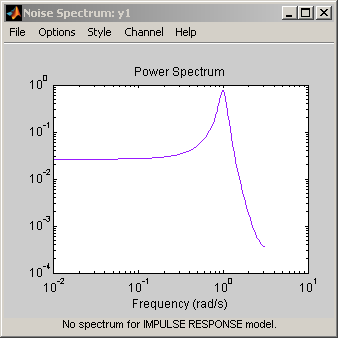## Plot the Noise Spectrum Using the System Identification App

To create a noise spectrum plot for parametric linear models in the app, select the Noise spectrum check box in the Model Views area. For general information about creating and working with plots, see Working with Plots.

To include or exclude a model on the plot, click the corresponding model icon in the System Identification app. Active models display a thick line inside the Model Board icon.

The following figure shows a sample Noise Spectrum plot.The following table summarizes the Noise Spectrum plot settings.

Noise Spectrum Plot Settings

ActionCommand

Display the confidence interval.

• To display the dashed lines on either side of the nominal model curve, select Options > Show confidence intervals. Select this option again to hide the confidence intervals.

• To change the confidence value, select Options > Set % confidence level, and choose a value from the list.

• To enter your own confidence level, select Options > Set confidence level > Other. Enter the value as a probability (between 0 and 1) or as the number of standard deviations of a Gaussian distribution.

Change the frequency values for computing the noise spectrum.

The default frequency vector is 128 linearly distributed values, greater than zero and less than or equal to the Nyquist frequency.

Select Options > Frequency range and specify a new frequency vector in units of radians per second.

Enter the frequency vector using any one of following methods:

• MATLAB® expression, such as `[1:100]*pi/100` or `logspace(-3,-1,200)`. Cannot contain variables in the MATLAB workspace.

• Row vector of values, such as `[1:.1:100]`

### Tip

To restore the default frequency vector, enter `[]`.

Change frequency units between hertz and radians per second.Select Style > Frequency (Hz) or Style > Frequency (rad/s).
Change frequency scale between linear and logarithmic.Select Style > Linear frequency scale or Style > Log frequency scale.
Change amplitude scale between linear and logarithmic.Select Style > Linear amplitude scale or Style > Log amplitude scale.

(Multiple-output system only)
Select an input-output pair to view the noise spectrum corresponding to those channels.

### Note

You cannot view cross spectra between different outputs.

Select the output by name in the Channel menu.
##### SupportGet trial now